# Angle at the centre of a circle is twice the angle at the circumference

By Martin McBride, 2023-02-15
Tags: circle chord angle
Categories: gcse geometryFor any chord, we can draw two lines to form an angle at the centre of the circle, angle b.

We can also draw two lines to form an angle at the circumference, a.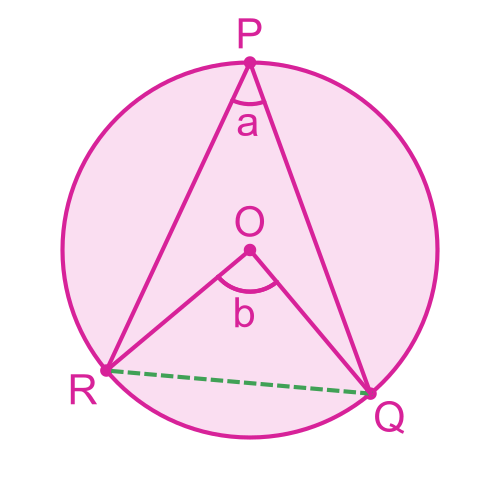The angle b formed at the centre of a circle is twice the angle a at the circumference.

Here is a video on this topic:

## Alternative shapes

If we move the point P around the circumference, the diagram looks different: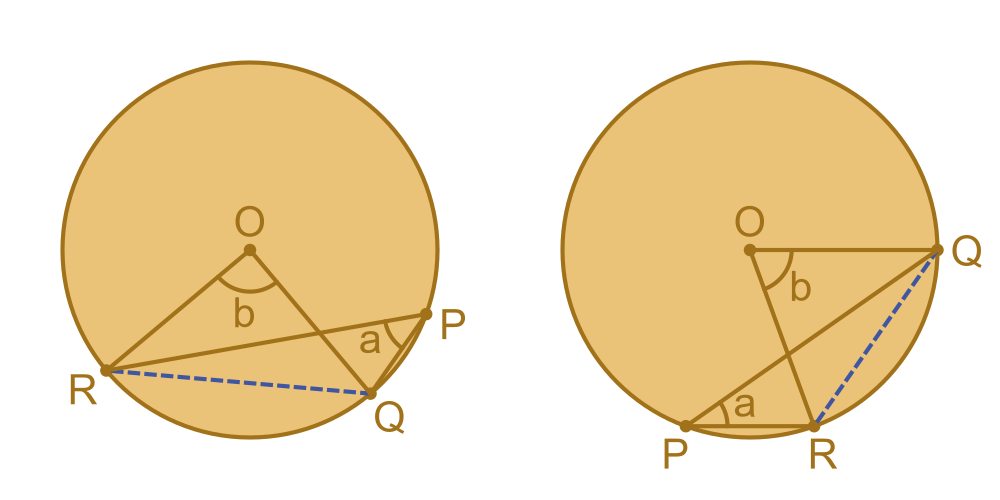Due to the position of P, the line PR crosses the line OQ. It is important to recognise that this is still the same situation, even though it looks a little different.

The chord RQ still forms an angle b at the centre, and an angle a at the circumference, and it is still true that angle b is twice angle a.

Here is another slightly different arrangement: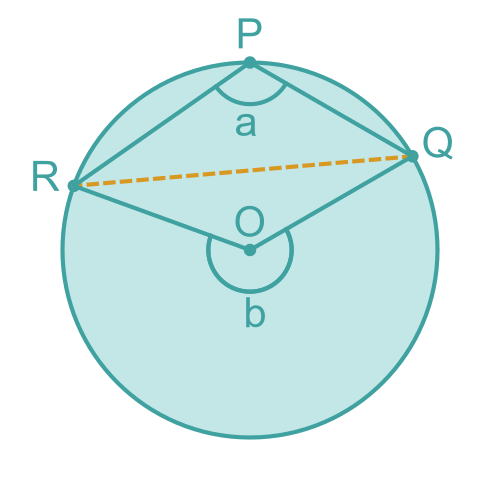This time the point P is in the minor sector formed by the lines OR and OQ.

The angle b is always in the opposite sector to the angle a. So in this case b is a reflex angle (an angle that is greater than 180°). Again, don't be confused by the different appearance of the diagram.

## Proof

The key to proving this result is to construct three triangles like this: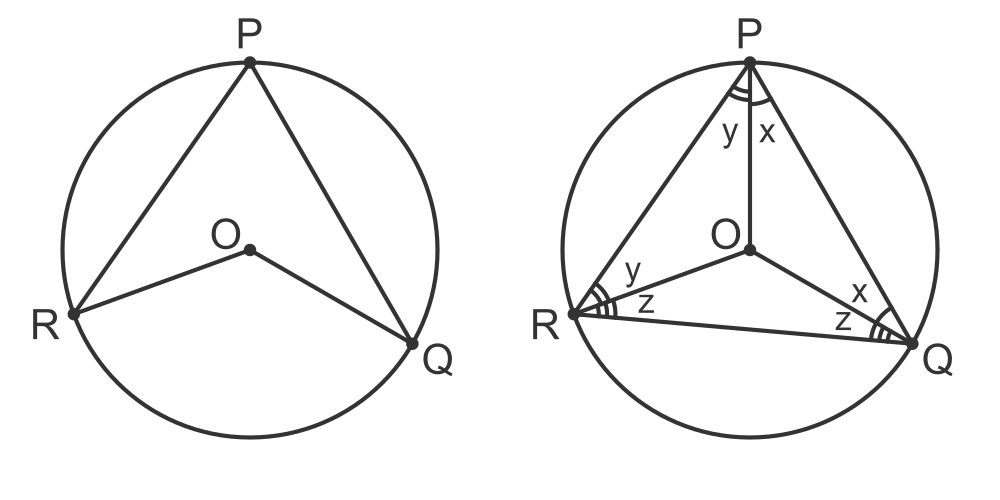Notice that OP, OQ and OR are all radii of the circle. There is a circle theorem that tells us that 2 radii form an isosceles triangle.

This means that:

• The two angles at the base of triangle PQO are equal, and we will call that angle x.
• The two angles at the base of triangle PRO are equal, and we will call that angle y.
• The two angles at the base of triangle QRO are equal, and we will call that angle z.

We can find the three angles of the triangle PQR, from the diagram:

• Angle P is x + y.
• Angle Q is x + z.
• Angle R is y + z.

Since we know that the three angles in a triangle add up to 180°, this gives us: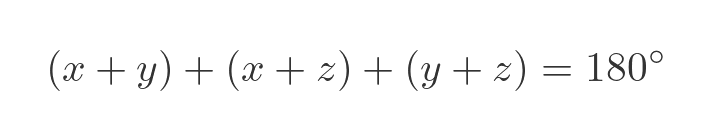This can be rearranged as: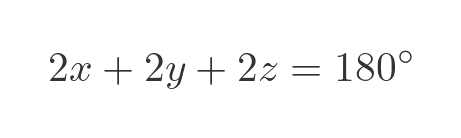Here is the triangle PQR redrawn to show the angle a: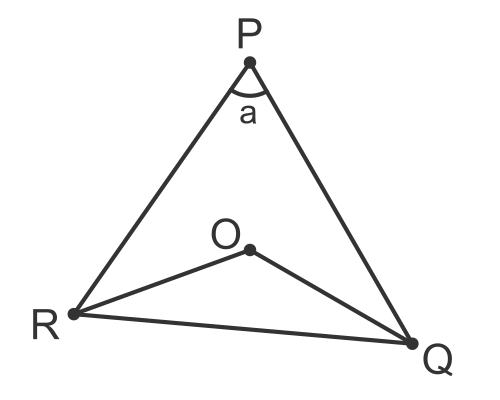We can see that the angle at a is equal to x + y from the previous diagram.

We can replace 2x + 2y with 2a in the earlier equation, to get: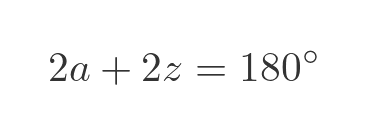Which means: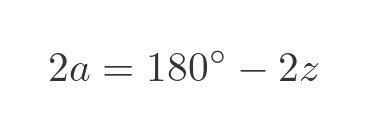Now let's look at the triangle QRO: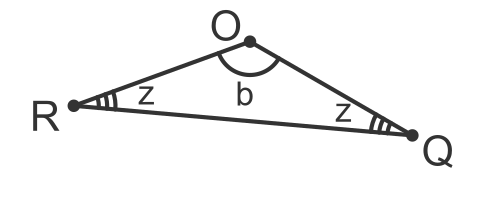For the earlier diagrams, we know that the angle at O is b, and the angles at Q and R are both equal to z. Since the angles in the triangle add up to 180° we can see that: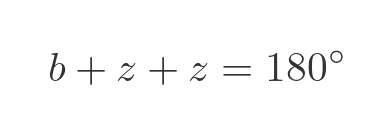We can solve for b: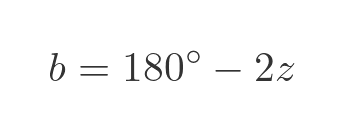Compare this with the previous value for 2a:This proves that angle b is twice angle a.